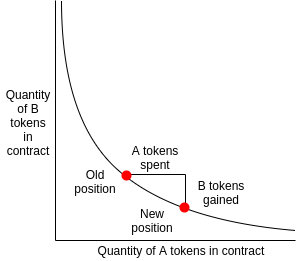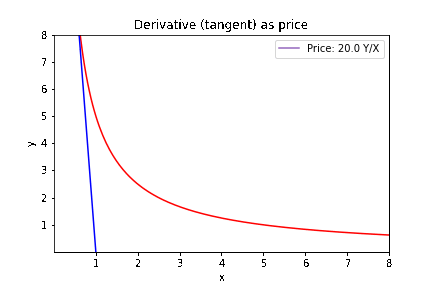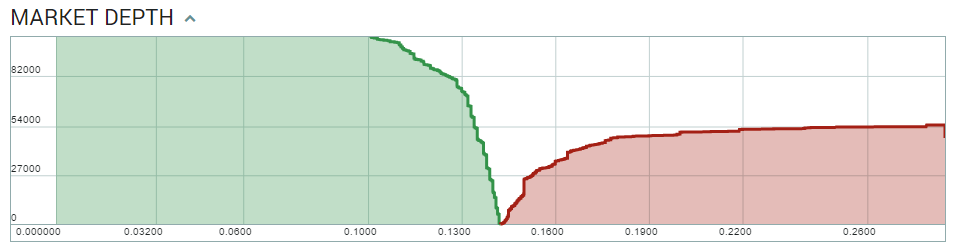8 Oct 2020

### A stablecoin exchange¶

Curve is an exchange designed for stablecoins and bitcoin tokens on Ethereum. The key aspect of Curve is its market-making algorithm, which can provide 100-1000 times higher market depth than Uniswap or Balancer for the same total value locked.

### Curve's Goals¶

The main goal is to let users and other decentralised protocols exchange stablecoins (DAI to USDC for example) through it with low fees and low slippage.

You can think of CURVE as a specialized version of the Uniswap exchange. To understand CURVE, we will examine how the Uniswap market making algorithm works. In this article we identify its weaknesses and limitations. Using the lessons learnt we generalize to pools with several coins and finally what problem CURVE is solving and how.

# 1. Uniswap's AMM algo¶

In its simplest form, Uniswap's pricing and trading algorithm can be summarised as:

$$x \cdot y = k$$Which means, that if the pool has x tokens of asset X and y tokens of asset Y, then the pool will update its assets such that before and after the trade, $x \cdot y = k$.

$k$ is called the invariant (invariant = thing that stays the same). For example:

there are 1000 X tokens and 5000 Y tokens $x \cdot y = 5 \times 10^6$

Somebody wants to buy Y tokens. To do so, they deposit 200 X tokens into the pool. After the 0.3% fee, 199.4 X tokens are added. The pool now has:

1199.4 X tokens.

The invariant $k = 5 \times 10^6$ has to stay the same, so: $1199.4 \cdot y = 5 \times 10^6$ must be true. We can therefore solve the equation and get: $y = 4168.75$ tokens. In other words, to preserve the invariant $k$, $y = 5000$ needs to go down to $y = 4169.75$. The difference $5000-4168.75 = 831.25$ is how many Y tokens the trader will receive.## Price Setting¶

You may have noticed that the price was automatically set. The trader deposited 200 X and got 831.25 Y, so the price is: $$\mathcal{P} = \frac{831.25}{200} \;\; Y/X$$

or $$\mathcal{P} = 4.16 \;\; Y/X$$

## Price Updating¶

What is the price if a new trader wants to trade another 200 X tokens for Y tokens? Well, let's apply $x \cdot y = k$ again to find out:

The new state of the pool after the deposit is:

(1199.4 + 199.4) X tokens

4168.75 Y tokens

So this time we need to solve $1398.8 \cdot y = 5 \times 10^6$. the solution is $y = 3574.4$, so the second trader will get (what_is_currently_in_the_Y_side - what_should_be_in_the_Y_side) $= 4168.75-3574 = 594.75$. As you see, the second trader got less for her money. The price was:

$$\mathcal{P} = 2.97 \;\; Y/X$$

Y got much more expensive after the first trade! In some ways this makes sense. In the first operation, somebody tried to buy $\frac{831}{5000} ~ 17\%$ of all Y tokens from the pool. In the face of such high demand, the pool auto-adjusted the price accordingly.

## Price Equation & geometrical interpretation¶

Notice that the price was calculated as $\mathcal{P} = \frac{\Delta y}{\Delta x}$. In other words,

$$\mathcal{P} = \frac{\textrm{how much was taken out}}{\textrm{how much was deposited}}$$

If we think of the $xy=k$ as a curve, the curve $y = \frac{k}{x}$, then $\mathcal{P} = \frac{\Delta y}{\Delta x}$ is its gradient or, in the limit of small trades, the derivative:

$$\mathcal{P} = \frac{d y}{d x}$$

This idea will come back later several times.In the graph above, as trading occurs, and we have less Y in the pool, but more X:

• if the tangent is shalow, you get less Y for your X (Y more expensive, low number)
• if the tangent is steep, you get more Y for your X (Y is cheaper)

## Slippage¶

In a standard exchange, the order-book:Allows us to read how the price will change (slippage) as we buy more and more of a token (travel up the curve). This depends on the details of the price and volume set by each trader. In the AMM, the $x \cdot y=k$ equation and depth of the pool determines this slippage.

For example, What if a single buyer had deposited 400 X tokens, instead of two subsequent buyers? This would have gotten us a price $\mathcal{P} = 3.56\;\; Y/X$, an average between the prices of the first and second buyer.

## Depth & Slippage¶

To find the slippage, we compare the price ($\mathcal{P}$) before and after the trade. Since the price is the derivative, we can say that if we exchange $\delta$ tokens of X the slippage is:

$$\frac{\left. \frac{dy}{dx}\right|_{x} - \left. \frac{dy}{dx}\right|_{x+\delta}}{\left. \frac{dy}{dx}\right|_{x}}$$

Since $\frac{dy}{dx} = \frac{-k}{x^2}$ for $y = \frac{k}{x}$, After substitution and rearrangement the slippage $\mathcal{S}$ is:

$$\mathcal{S} = 1 - \left(\frac{x}{x+\delta}\right)^2$$

To interpret this formula we can look at two obvious limits:

1. If we buy a small amount of X, then $\delta << x$, so $\frac{x}{x+\delta}$ approaches $1$, and slippage tends to zero.

2. For a deep pool, $x \rightarrow \infty$, so slippage also approaches 0.

Therefore, to avoid price swings, deep liquidity is required. If we rework the numbers with:

• X as 1M tokens
• Y has 5M tokens
• $k = 5 \times 10^{12}$

and deposit X tokens a few times, you can see that the price will be very stable:

Trade Nr # of X exchanged Price (Y/X)
1 10 4.9849
2 10 4.9848
3 10 4.9847

or even:

Trade Nr # of X exchanged Price (Y/X)
1 10,000 4.9
2 10,000 4.8
3 10,000 4.7

The initial price, of course is given by the initial $\frac{y}{x} = 5$ ratio (gradient at x = 1M).

## Arbitrage & Equilibrium¶

In an efficient market, however, traders will spot these arbitrage opportunities. Just like the price of Y was increasing, the price of X (denominated in Y) will be decreasing. Arbitrageurs will buy Y elsewhere and try to sell it in this pool (or buy X in the pool, and sell it, for example in a high volume centralized exchange). For a token with high liquidity elsewhere the short term dynamics may look more like so: